# Graphical representation of the weak mixing angle

• B
Sandglass
The graphical pattern of particles in the weak hypercharge and weak isospin plane, visible in this wiki page, shows the mixing angle between the Yw and Q axes. Actually , from the weak hypercharge (-2) of a right-handed electron and its electric charge (-1), one obtains an angle Pi/3, not the mixing angle.

So is this mixing angle a symbolic representation of the link between electromagnetism and the weak interaction, derived from the complex calculations of the standard model, or does it have a numerical use in this graph?

Gold Member
Not a complete answer, but the numerical applications of electroweak unification primarily derive from the other diagram in the same article, which demonstrate that the electromagnetic force coupling constant, the weak force coupling constant, and the Weinberg angle are not independent of each other.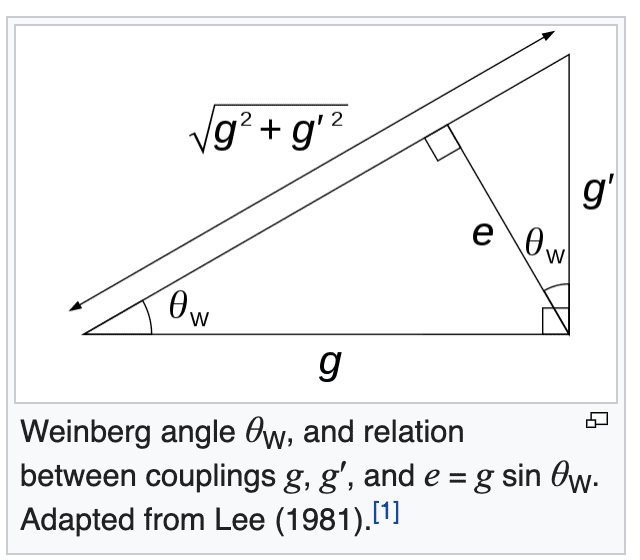Likewise, the W boson mass, the Z boson mass, and the Weinberg angle are not independent of each other, and the relative magnitudes of those Standard Model constants are functions of the electromagnetic and weak force coupling constants. In other words: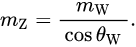and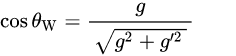and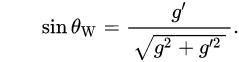and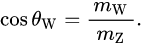So, while we have five parameters (the Weinberg angle, the electromagnetic coupling constant, the weak force coupling constant, the W boson mass and the Z boson mass), because of electroweak unification, those five parameters don't represent five independent degrees of freedom.

The original W0 and B0 vector bosons of electroweak unification theory are really more of a conceptual framework than something that physicists work with to solve problems, or observe, that illustrates a way of thinking about how the relationships that are observed arise.

•topsquark and Sandglass
Sandglass

Since the coupling constant for the weak hypercharge is given by g' cos (theta_w) = e, I naively thought that the weak hypercharge Yw was related to the electric charge Q in the same way by Yw cos(theta_w) = Q. What puzzles me is the fact that the particle graph seems to confirm this with a mixing angle shown in the (Yw, Q) plane.

On the other hand, this cannot be the case since the Gell-Mann-Nishijima formula Q = Yw/2 + T3 cannot be satisfied with g'/e = Yw/Q = cos(theta_w) and g/e = T3/Q = sin(theta_w).

Therefore, is there any theoretical relationship between (g'/e) and (Yw/Q) ?

•ohwilleke
Sandglass
I'm making one last attempt about this graph. I looked for it in some QFT books and didn't see it.

Does anyone have a reference, either an academic book or a review article that shows this graph, instead of this wiki page ?

Gold Member
2022 Award
I don't know. Why is it important? It just depicts the relation between the coupling constants ##g##, ##g'## and ##e## in terms of the Weinberg angle. Wikipedia gives a source for the picture:

Lee, T.D. (1981). Particle Physics and Introduction to Field Theory.

It's a nice textbook by a Nobel laureate.

•ohwilleke
Sandglass
•ohwilleke
Gold Member
do the letters L and R for particles refer to chirality, not helicity? The legend is ambiguous.
Fermions have chirality. Bosons have helicity. All of the references in the chart with L or R that I see are for fermions, so it is a reference to chirality.

Homework Helper
Gold Member
2022 Award
Fermions have chirality. Bosons have helicity. All of the references in the chart with L or R that I see are for fermions, so it is a reference to chirality.
Not enitirely true. Chirality is a label for how the particle transforms under the Lorentz group.

Helicity is never used as a label for anything in these kind of diagrams, since it is not a Lorentz invariant property of the particle.

•Gold Member
2022 Award
Fermions have chirality. Bosons have helicity. All of the references in the chart with L or R that I see are for fermions, so it is a reference to chirality.
Dirac fermions have indeed both chirality and helicity. By definition chirality eigenstates are eigenstates of ##\gamma_5##. Since ##\gamma_5^2=1## the possible eigenvalues of chirality are ##\chi \in \{1,-1 \}##.

By definition helicity for a momentum eigenstate is the eigenvalue of the component of the total angular momentum in direction of the momentum, and the corresponding eigenvalues are ##h \in \{1/2,-1/2\}## (with natural units, where ##\hbar=1##).

Only for massless particles ##\chi=2h##, and only in this case helicity eigenstates are Lorentz invariant. For massive particles you can change to another reference frame, where helicity is flipped. That's impossible for massless particles, because you cannot "overtake" the fermion with your new reference frame, because a massless fermion moves with ##c## in all inertial frames.

•LittleSchwinger and ohwilleke
Gold Member
Thanks for the corrections that have clarified the concepts.

Homework Helper
Gold Member
2022 Award
Furthermore, when we use the labels R and L on fermions in the electroweak SM we are referring to chirality always - the unbroken EW symmetry. This is because they L and R chirality have different gauge couplings.

•LittleSchwinger, ohwilleke and vanhees71
Sandglass
Thanks for all these clarifications.

Gold Member
$$Q e = Y_W \frac{1}{2} g' \cos{\theta_W} + T_3 g \sin{\theta_W}$$
•